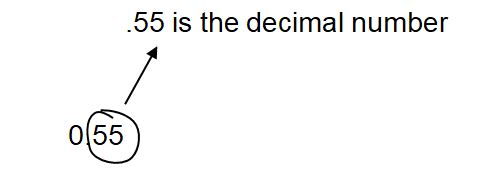###### Latest Topics# An overview of the decimal form:-

A decimal number can be expressed by a fraction with a power of ten. For instance, the decimal form of the fraction 5/10 is 0.5, where:

Consider the decimal number 0.55.The decimal point is denoted by a dot.

To the right of the decimal point, there is the fractional part. Here,

The number immediately before the decimal point indicates the whole number component of the friction. Here, the whole number is zero.

Similarly, consider 5.95. Here 5 is the whole number and 0.95 is the decimal number.Application of decimal numbers in real life:-

Money: Rs. 5.50 means 5 rupees and fifty paise. 5 is the whole number (rupees) and 0.50 is the decimal number (paise).

Length: 5.5 meters means 5 (whole number) meters and 0.5 (or half) meters, which is the decimal number.

Temperature: 30.5 degrees.  Here 30 is the temperature in the whole number and 0.5 degrees is the temperature in decimal number.

Mass: 99.5 kg.

Adding or subtracting decimals is similar to adding or subtracting whole numbers.

When we add fractions, we add the parts of the whole number and the parts of the fraction separately. If necessary, the 1 is carried from the fractional part to the whole number part.  The decimal points of decimals are aligned so as to line up the whole number parts and the decimal parts.  Then, they are added.

Step 1:  Align the numbers vertically initially. It should be done in such a way that the decimal points are aligned on one line. All whole numbers will come on the left and decimal numbers after the DOT on the right.

Example:

Step 2: Start the addition with numbers on the extreme right, that is, the decimal numbers. Then carry forward the first number to the left if it’s a two-digit number after addition.

Example:

1->carry forward number

45.55

+20.05

_______

65.60.

0 . 5  5

0.  0  5

Add 5+5 = 10, where 1 is the carry forward number, which lands on the top of 5, as shown below:

1->carry forward number

45.55

+20.05

Now, add 1+5+0 = 6. These numbers are underlined in the addition shown below:

1->carry forward number

45.55

+20.05

Example:

1->carry forward number

45.55

+20.05

_______

65.60.

Then, add the whole number on the left. Those are, 45 + 20 = 65

Subtraction of decimal numbers:

Let us consider the numbers as before.

45.55

- 20.05

_______

Here initially, we start with the subtraction of 0.55 and 0.05. Starting from the extreme right:

0 . 5  5

0.  0  5

Add 5-5 = 0 and next 5-0 = 0, as shown below:

45.55

- 20.05

_______

.50

Next move over to the right. 5-0 =0 and 4-2 = 2. Therefore, the whole number part is  45-20=25.

45.55

- 20.05

_______

25.50